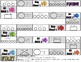# 3rd Grade Math Centers Module 5 Eureka Math BUNDLESubject
Resource Type
File Type

PDF

(8 MB|19 pages)
Product Rating
Standards
• Product Description
• StandardsNEW

**Purchase the ENTIRE 3rd Grade Eureka Math Modules 1-7 BUNDLE here-

3rd Grade Math Centers Module 5 Eureka Math BUNDLE

7 Fun Centers Included-

1. Pizza Parts Cootie Catcher: Represent parts of one whole as fractions with number bonds

2. Memory Match: Vocabulary practice for Module 5

3. Tic Tac Toe: Identify shaded fractional parts in different ways depending on the designation of the whole

4. Roll & Record: Express whole numbers as fractions and recognize equivalence with different units

5. Fractional Fish Board Game: Represent & identify fractional parts of different wholes

6. Shake & Solve: Understand distance and position on the number line as strategies fo comparing fractions

7. QR Code Math- Compare fractions with the same numerator using <, > or = & use a model to reason about their size

-It would mean so much to me if you would leave feedback..even one or two words! Thanks so much!!! :)

Partition shapes into parts with equal areas. Express the area of each part as a unit fraction of the whole. For example, partition a shape into 4 parts with equal area, and describe the area of each part as 1/4 of the area of the shape.
Compare two fractions with the same numerator or the same denominator by reasoning about their size. Recognize that comparisons are valid only when the two fractions refer to the same whole. Record the results of comparisons with the symbols >, =, or <, and justify the conclusions, e.g., by using a visual fraction model.
Express whole numbers as fractions, and recognize fractions that are equivalent to whole numbers. Examples: Express 3 in the form 3 = 3/1; recognize that 6/1 = 6; locate 4/4 and 1 at the same point of a number line diagram.
Recognize and generate simple equivalent fractions, (e.g., 1/2 = 2/4, 4/6 = 2/3). Explain why the fractions are equivalent, e.g., by using a visual fraction model.
Understand two fractions as equivalent (equal) if they are the same size, or the same point on a number line.
Total Pages
19 pages
Included
Teaching Duration
N/A
Report this Resource to TpT
Reported resources will be reviewed by our team. Report this resource to let us know if this resource violates TpT’s content guidelines.